# Symmetry

###### Question 2.For the given figure, which one is the mirror line, l1l1 or l2?l2?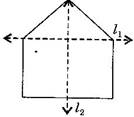Answer: l2l2 is the mirror line as both sides of the lines are symmetric.

NCERT Solutions for Class 6 Maths Exercise 13.1

###### Question 3.Identify the shapes given below. Check whether they are symmetric or not. Draw the line of symmetry as well.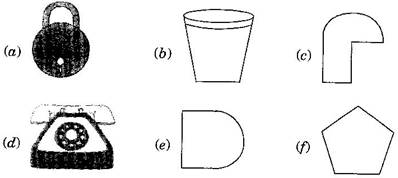(a) Symmetric(b) Symmetric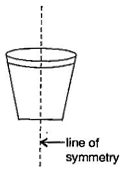(c) Not symmetric

(d) Symmetric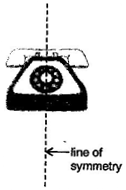(e) Symmetric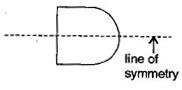(f)SymmetricNCERT Solutions for Class 6 Maths Exercise 13.1

###### Question 4.Copy the following on a square paper. A square paper is what you would have used in your arithmetic notebook in earlier classes. Then complete them such that the dotted line is the line of symmetry.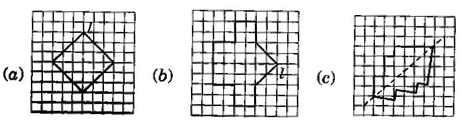###### Question 5.In the figure, ll is the line of symmetry. Complete the diagram to make it symmetric.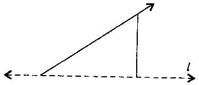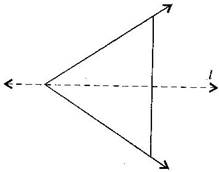NCERT Solutions for Class 6 Maths Exercise 13.1

###### Question 6.In the figure, ll is the line of symmetry. Draw the image of the triangle and complete the diagram, so that it becomes symmetric.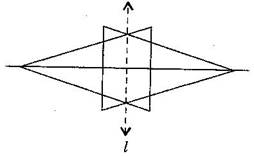###### Question 1.Find the number of lines of symmetry for each of the following shapes: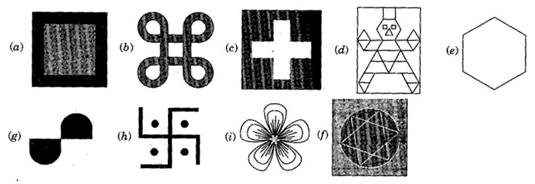(a) 4, (b) 4, (c) 4, (d) 1, (e) 6, (f) 4, (g) 0, (h) 0, (i) 3

###### Question 2. Copy the triangle in each of the following figures, on squared paper. In each case, draw the line(s) of symmetry. If any and identity the type of triangle. (Some of you may like to trace the figures and try paper-folding first!)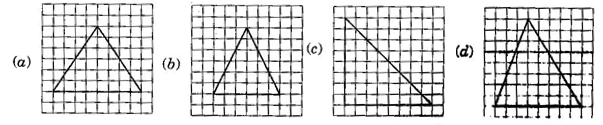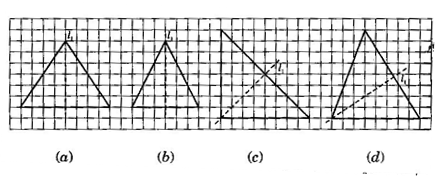(a) l1l1 is the line of symmetry.

(b) l1l1 is the line of symmetry.

(c) l1l1 is the line of symmetry.

(d) No line of symmetry.

NCERT Solutions for Class 6 Maths Exercise 13.2

###### Question 3.Complete the following table:
 Shape Rough figure No. of lines of symmetry Equilateral triangle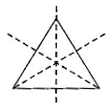3 Square Rectangle Isosceles triangle Rhombus Circle

 Shape Rough figure No. of lines of symmetry Equilateral triangle3 Square4 Rectangle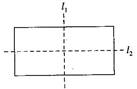2 Isosceles triangle1 Rhombus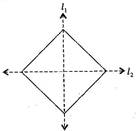2 CircleInfinite

NCERT Solutions for Class 6 Maths Exercise 13.2

###### Question 4.Can you draw a triangle which has:

(a) exactly one line of symmetry?

(b) exactly two lines of symmetry?

(c) exactly three lines of symmetry?

(d) no lines of symmetry?

Sketch a rough figure in each case.

(a) Yes, Isosceles triangle(b) No such triangle cannot be formed.

(c) Yes, Equilateral triangle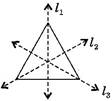(d) Yes, Scalene triangle###### Question 5.On a squared paper, sketch the following:

(a) A triangle with a horizontal line of symmetry but no vertical line of symmetry.

(b) A quadrilateral with both horizontal and vertical lines of symmetry.

(c) A quadrilateral with a horizontal line of symmetry but no vertical line of symmetry.

(d) A hexagon with exactly with two lines of symmetry.

(e) A hexagon with six lines of symmetry.

(Hint: It will be helpful if you first draw the lines of symmetry and then complete the figures)

(a)(b)(c)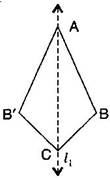(d)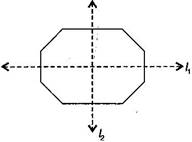(e)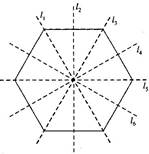NCERT Solutions for Class 6 Maths Exercise 13.2

###### Question 6.Trace each figure and draw the lines of symmetry, if any: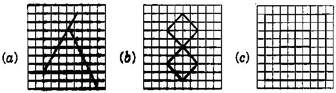(a) No line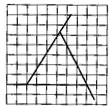(b) Two lines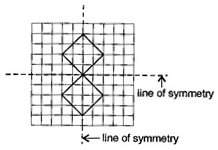(c) Four lines(d) Two lines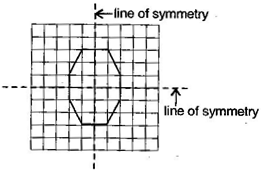(e) One line(f)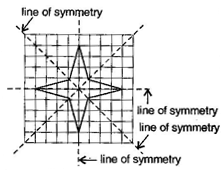###### Question 7.Consider the letters of English alphabets A to Z. List among them the letters which have:

(a) vertical lines of symmetry (like A)

(b) horizontal lines of symmetry (like B)

(c) no lines of symmetry (like Q)Vertical lines: A, H, I, M, O, T, U, V, W, X, Y

Horizontal lines: B, C, D, E, H, I, K, O, X

No line of symmetry: F, G, J, I, N, P, Q, R, S, Z

NCERT Solutions for Class 6 Maths Exercise 13.2

###### Question 8.Given here are figures of a few folded sheets and designs drawn about the fold. In each case, draw a rough diagram of the complete figure that would be seen when the design is cut off.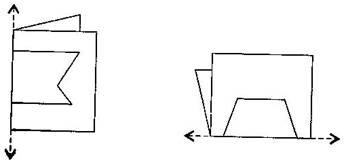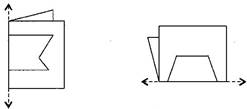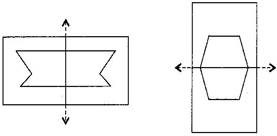###### Question 1.Find the number of lines of symmetry in each of the following shapes. How will you check your answer?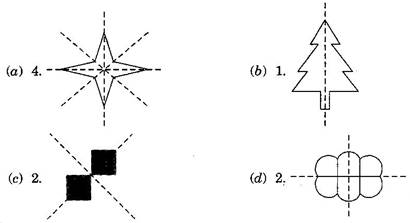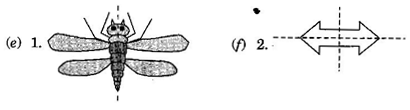NCERT Solutions for Class 6 Maths Exercise 13.3

###### Question 2.Copy the following drawing on squared paper. Complete each one of them such that the resulting figure has two dotted lines as two lines of symmetry.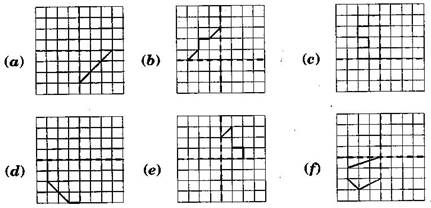How did you go about completing the picture?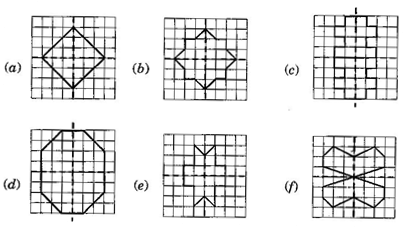NCERT Solutions for Class 6 Maths Exercise 13.3

###### Question 3.In each figure below, a letter of alphabet is shown along with a vertical line. Take the mirror image of the letter in the given line. Find which letters look the same after reflection (i.e., which letters look the same in the image) and which do not. Can you guess why?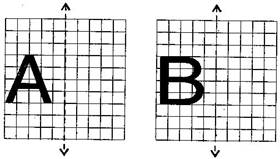Try for O E M N P H L T S V X

Same after reflection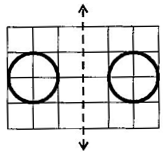Different after reflectionSame after reflectionDifferent after reflectionDifferent after reflection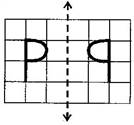Same after reflectionDifferent after reflection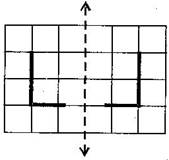Same after reflection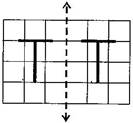Different after reflectionSame after reflection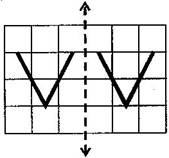Same after reflection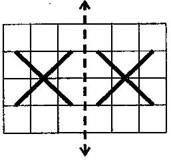error: Content is protected !!